# Selina Concise Mathematics Class 9 ICSE Solutions Circle

## Selina Concise Mathematics Class 9 ICSE Solutions Circle

APlusTopper.com provides step by step solutions for Selina Concise Mathematics Class 9 ICSE Solutions Chapter 17 Circle. You can download the Selina Concise Mathematics ICSE Solutions for Class 9 with Free PDF download option. Selina Publishers Concise Mathematics for Class 9 ICSE Solutions all questions are solved and explained by expert mathematic teachers as per ICSE board guidelines.

Selina ICSE Solutions for Class 9 Maths Chapter 17 Circle

Exercise 17(A)

Solution 1: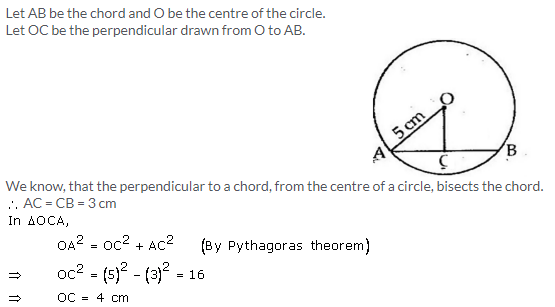Solution 2:Solution 3:Solution 4: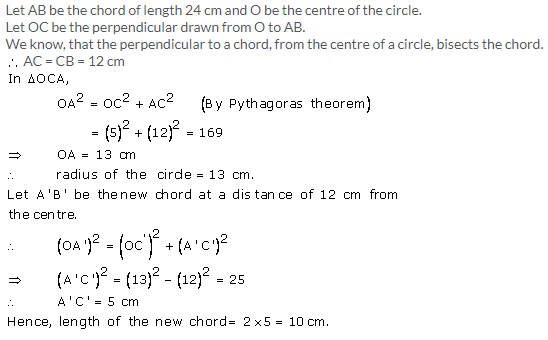Solution 5:Solution 6:
Let O be the centre of the circle and AB and CD be the two parallel chords of length 30 cm and 16 cm respectively.
Drop OE and OF perpendicular on AB and CD from the centre O.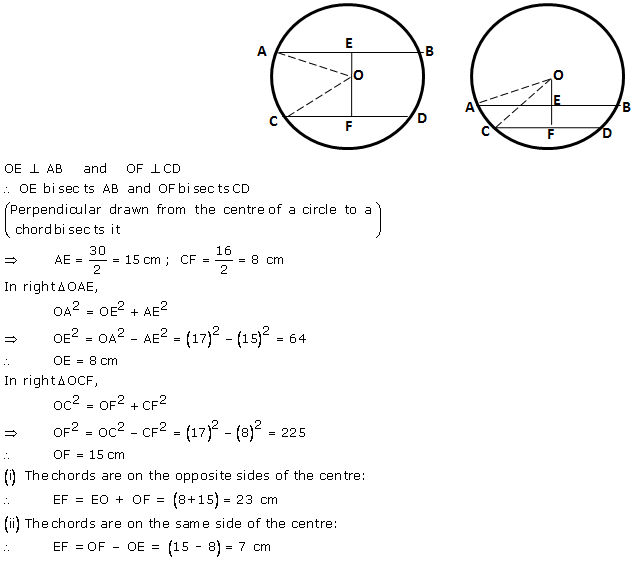Solution 7:
Since the distance between the chords is greater than the radius of the circle (15 cm), so the chords will be on the opposite sides of the centre.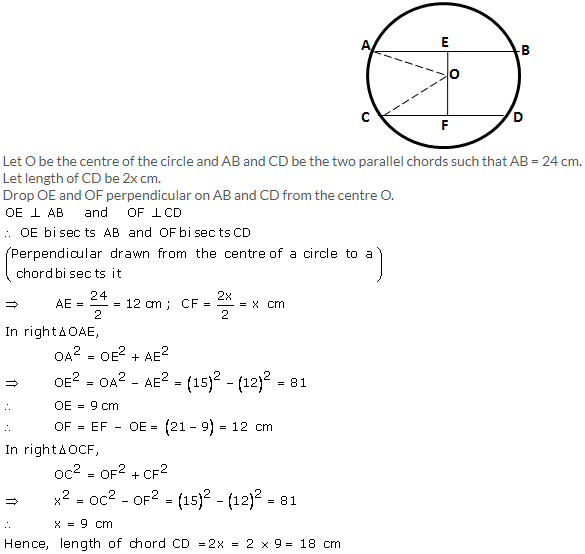Solution 8: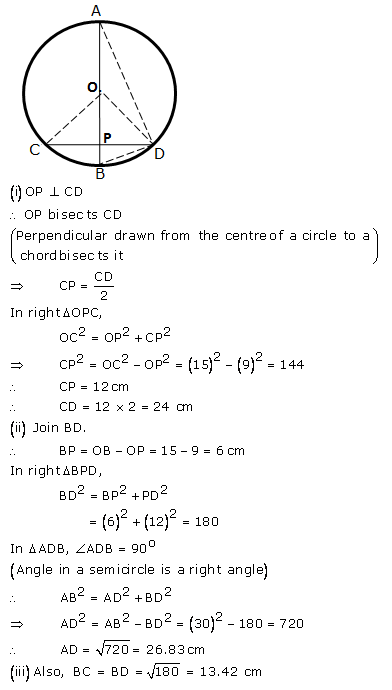Solution 9:Solution 10: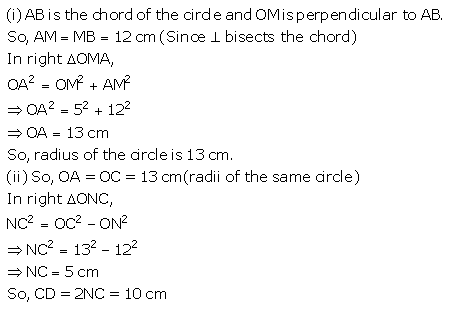Exercise 17(B)

Solution 1:Solution 2:Solution 3:Solution 4: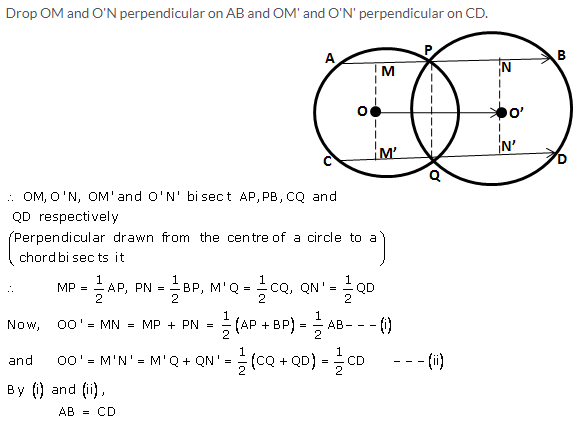Solution 5:Solution 6:Solution 7:Solution 8: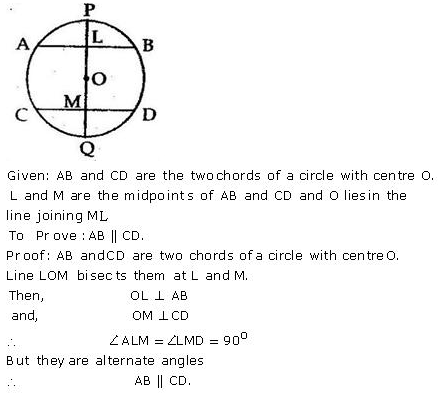Solution 9:Solution 10:Exercise 17(C)

Solution 1: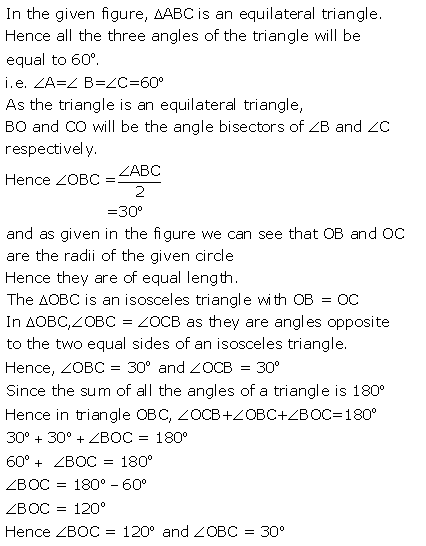Solution 2: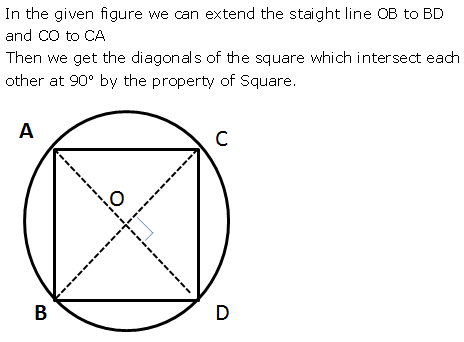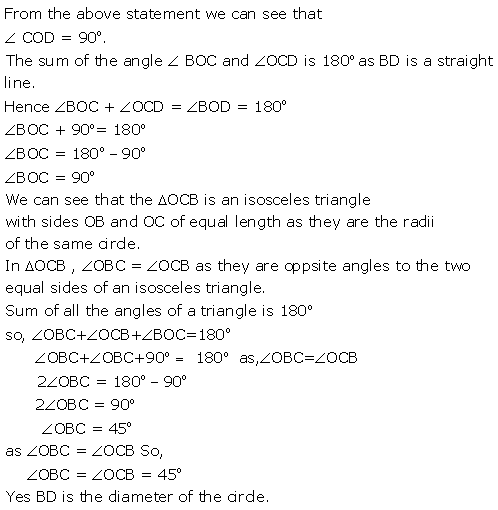Solution 3:
As given that AB is the side of a pentagon the angle subtended by each arm of the pentagon atSolution 4: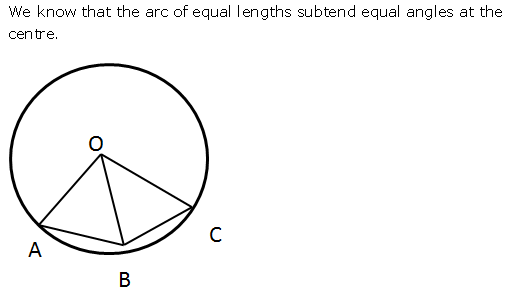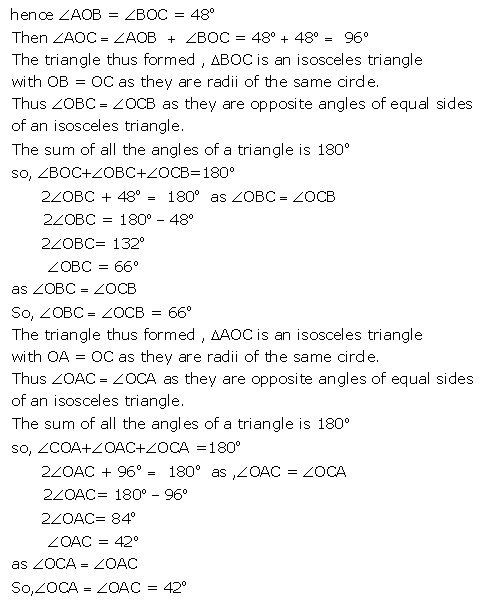Solution 5: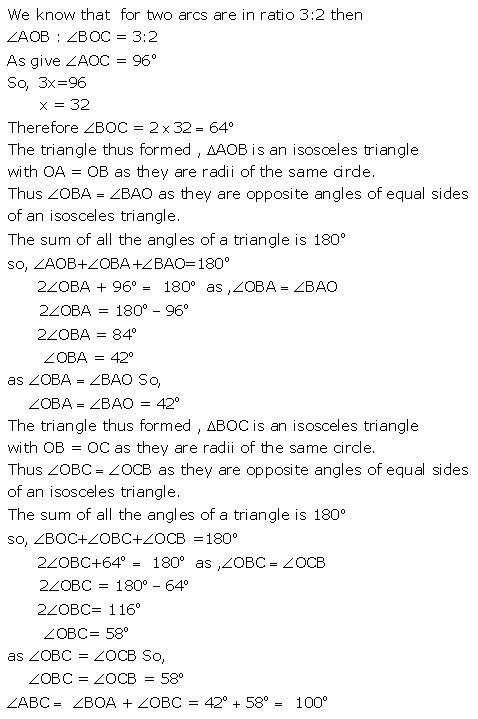Solution 6: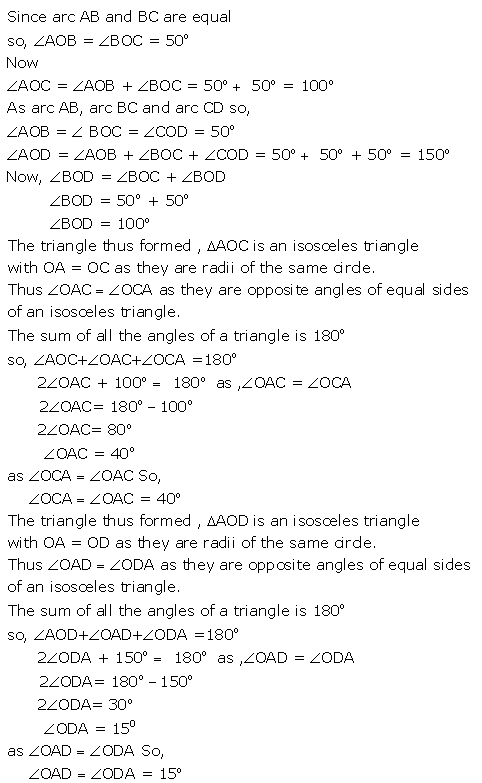Solution 7:Solution 8:Exercise 17(D)

Solution 1: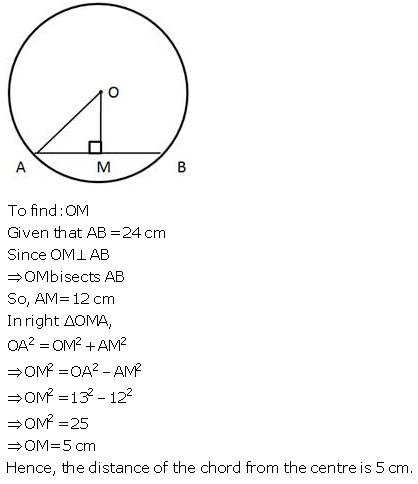Solution 2: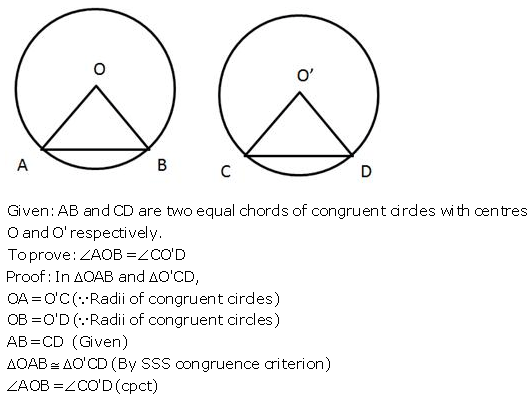Solution 3:Solution 4: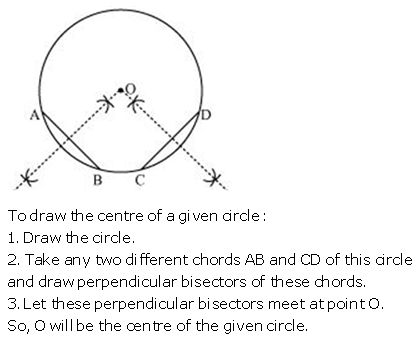Solution 5:Solution 6:Solution 7:Solution 8:Solution 9: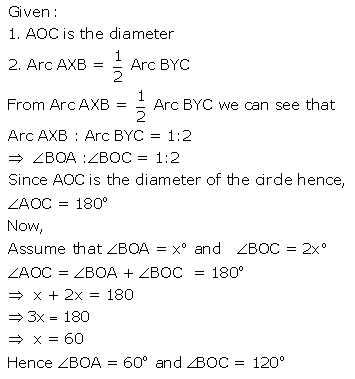Solution 10:More Resources for Selina Concise Class 9 ICSE Solutions